## The Law of Reflection and Its Consequences

The law of reflection states that the angle of reflection equals the angle of incidence.

### Learning Objectives

Formulate the relationship between the angle of reflection and the angle of incidence

### Key Takeaways

#### Key Points

• Light strikes different parts of a rough surface at different angles and is reflected, or diffused, in many different directions.
• A mirror has a smooth surface (compared with the wavelength of light) and so reflects light at specific angles.
• We see the light reflected off a mirror coming from a direction determined by the law of reflection.

#### Key Terms

• reflection: the property of a propagated wave being thrown back from a surface (such as a mirror)

Whenever you look into a mirror or squint at sunlight glinting off a lake, you are seeing a reflection. When you look at the text in a book, you are actually seeing the light that is reflected from it. Large telescopes use reflections to form images of stars and other astronomical objects. In fact, the only way we can see an object that does not itself emit light is if that object reflects light.

The law of reflection is illustrated in, which also shows how the angles are measured relative to the perpendicular to the surface at the point where the light ray strikes. The law of reflection is very simple: The angle of reflection equals the angle of incidence. When we see our reflection in a mirror, it appears that our image is actually behind the mirror — we see the light coming from a direction determined by the law of reflection. The angles are such that our image appears exactly the same distance behind the mirror as we stand away from the mirror.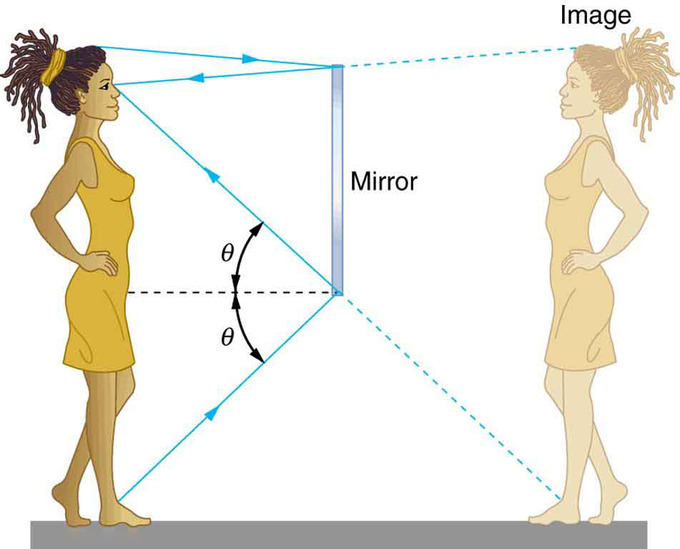Mirror Reflection: An image in a mirror appears as though it is behind the mirror. The two rays shown are those that strike the mirror at just the correct angles to be reflected into the eyes of the viewer. The image appears to come from the direction the rays are coming from when they enter the viewer’s eyes.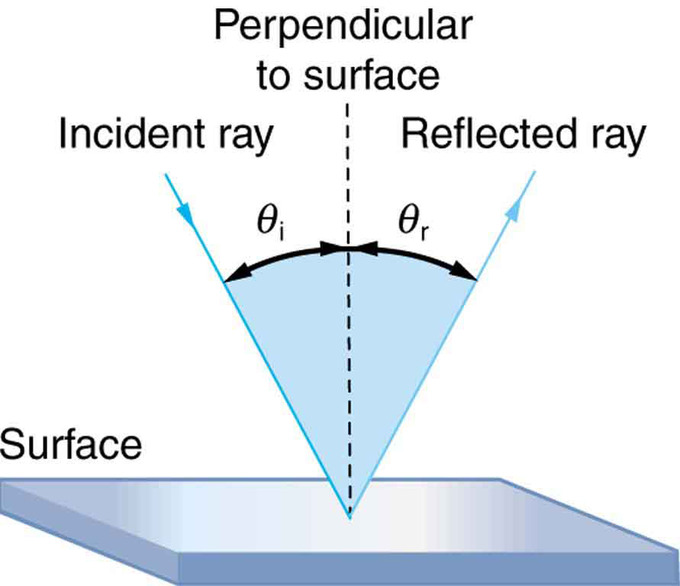Law of Reflection: The law of reflection states that the angle of reflection equals the angle of incidence: θr = θi. The angles are measured relative to the perpendicular to the surface at the point where the ray strikes the surface.

We expect to see reflections off a smooth surface. However, light strikes different parts of a rough surface at different angles, and it is reflected in many different directions (“diffused”). Diffused light is what allows us to see a sheet of paper from any angle. Many objects, such as people, clothing, leaves, and walls, have rough surfaces and can be seen from all sides. A mirror, on the other hand, has a smooth surface (compared with the wavelength of light) and reflects light at specific angles. When the moon reflects off the surface of a lake, a combination of these effects takes place.

Reflection: A brief overview of reflection and the law of reflection.

## The Law of Refraction: Snell’s Law and the Index of Refraction

The amount that a light ray changes its direction depends both on the incident angle and the amount that the speed changes.

### Learning Objectives

Formulate the relationship between the index of refraction and the speed of light

### Key Takeaways

#### Key Points

• The changing of a light ray’s direction (loosely called bending) when it passes through variations in matter is called refraction.
• The index of refraction is n=c/v, where v is the speed of light in the material, c is the speed of light in vacuum, and n is the index of refraction.
• Snell’s law, the law of refraction, is stated in equation form as $\text{n}_1\sin{θ_1}=\text{n}_2\sin{θ_2}$.

#### Key Terms

• refraction: Changing of a light ray’s direction when it passes through variations in matter.
• index of refraction: For a material, the ratio of the speed of light in vacuum to that in the material.

It is easy to notice some odd things when looking into a fish tank. For example, you may see the same fish appearing to be in two different places. This is because light coming from the fish to us changes direction when it leaves the tank, and in this case, it can travel two different paths to get to our eyes. The changing of a light ray’s direction (loosely called bending) when it passes through variations in matter is called refraction. Refraction is responsible for a tremendous range of optical phenomena, from the action of lenses to voice transmission through optical fibers.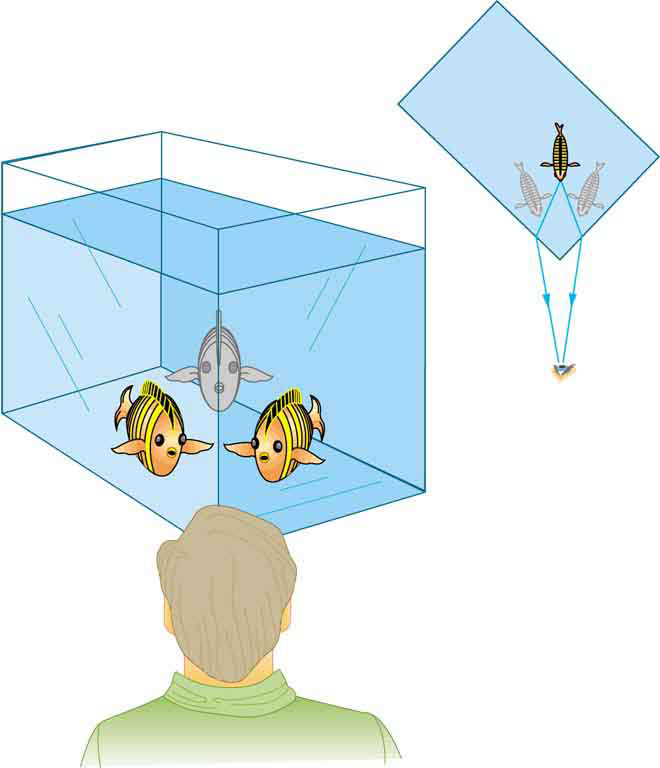Law of Refraction: Looking at the fish tank as shown, we can see the same fish in two different locations, because light changes directions when it passes from water to air. In this case, the light can reach the observer by two different paths, and so the fish seems to be in two different places. This bending of light is called refraction and is responsible for many optical phenomena.

Refraction: The changing of a light ray’s direction (loosely called bending) when it passes through variations in matter is called refraction.

### Speed of Light

The speed of light c not only affects refraction, it is one of the central concepts of Einstein’s theory of relativity. The speed of light varies in a precise manner with the material it traverses. It makes connections between space and time and alters our expectations that all observers measure the same time for the same event, for example. The speed of light is so important that its value in a vacuum is one of the most fundamental constants in nature as well as being one of the four fundamental SI units.

Why does light change direction when passing from one material ( medium ) to another? It is because light changes speed when going from one material to another.

### Law of Refraction

A ray of light changes direction when it passes from one medium to another. As before, the angles are measured relative to a perpendicular to the surface at the point where the light ray crosses it. The change in direction of the light ray depends on how the speed of light changes. The change in the speed of light is related to the indices of refraction of the media involved. In mediums that have a greater index of refraction the speed of light is less. Imagine moving your hand through the air and then moving it through a body of water. It is more difficult to move your hand through the water, and thus your hand slows down if you are applying the same amount of force. Similarly, light travels slower when moving through mediums that have higher indices of refraction.

The amount that a light ray changes its direction depends both on the incident angle and the amount that the speed changes. For a ray at a given incident angle, a large change in speed causes a large change in direction, and thus a large change in angle. The exact mathematical relationship is the law of refraction, or “Snell’s Law,” which is stated in equation form as:

n1sinθ1 = n2sinθ2

Here n1 and n2 are the indices of refraction for medium 1 and 2, and θ1 and θ2 are the angles between the rays and the perpendicular in medium 1 and 2. The incoming ray is called the incident ray and the outgoing ray the refracted ray, and the associated angles the incident angle and the refracted angle. The law of refraction is also called Snell’s law after the Dutch mathematician Willebrord Snell, who discovered it in 1621. Snell’s experiments showed that the law of refraction was obeyed and that a characteristic index of refraction n could be assigned to a given medium.

Understanding Snell’s Law with the Index of Refraction: This video introduces refraction with Snell’s Law and the index of refraction.The second video discusses total internal reflection (TIR) in detail. http://www.youtube.com/watch?v=fvrvqm3Erzk

## Total Internal Reflection and Fiber Optics

Total internal reflection happens when a propagating wave strikes a medium boundary at an angle larger than a particular critical angle.

### Learning Objectives

Formulate conditions required for the total internal reflection

### Key Takeaways

#### Key Points

• The critical angle is the angle of incidence above which total internal reflection occurs and given as $\theta_\text{c} = \arcsin \left( \frac{\text{n}_2}{\text{n}_1} \right)$.
• The critical angle is only defined when n2/n1 is less than 1.
• If light is incident on an optical fiber with an angle of incidence greater than the critical angle then the light will remain trapped inside the glass strand. Light can travel over a very long distance without a significant loss.

#### Key Terms

• Snell’s law: A formula used to describe the relationship between the angles of incidence and refraction.
• cladding: One or more layers of materials of lower refractive index, in intimate contact with a core material of higher refractive index.

Total internal reflection is a phenomenon that happens when a propagating wave strikes a medium boundary at an angle larger than a particular critical angle with respect to the normal to the surface. If the refractive index is lower on the other side of the boundary and the incident angle is greater than the critical angle, the wave cannot pass through and is entirely reflected. The critical angle is the angle of incidence above which the total internal reflectance occurs.

What is Total Internal Reflection?: Describes the concept of total internal reflection, derives the equation for the critical angle and shows one example.

### Critical angle

The critical angle is the angle of incidence above which total internal reflection occurs. The angle of incidence is measured with respect to the normal at the refractive boundary (see diagram illustrating Snell’s law ). Consider a light ray passing from glass into air. The light emanating from the interface is bent towards the glass. When the incident angle is increased sufficiently, the transmitted angle (in air) reaches 90 degrees. It is at this point no light is transmitted into air. The critical angle $\theta_\text{c}$ is given by Snell’s law, $\text{n}_1\sin\theta_1 = \text{n}_2\sin\theta_2$. Here, n1 and n2 are refractive indices of the media, and $\theta_1$ and $\theta_2$are angles of incidence and refraction, respectively. To find the critical angle, we find the value for $\theta_1$ when $\theta_2$= 90° and thus $\sin \theta_2 = 1$. The resulting value of $\theta_1$ is equal to the critical angle $\theta_\text{c} = \theta_1 = \arcsin \left( \frac{\text{n}_2}{\text{n}_1} \right)$. So the critical angle is only defined when n2/n1 is less than 1.Fig 1: Refraction of light at the interface between two media, including total internal reflection.

### Optical Fiber

Total internal reflection is a powerful tool since it can be used to confine light. One of the most common applications of total internal reflection is in fibre optics. An optical fibre is a thin, transparent fibre, usually made of glass or plastic, for transmitting light. The construction of a single optical fibre is shown in.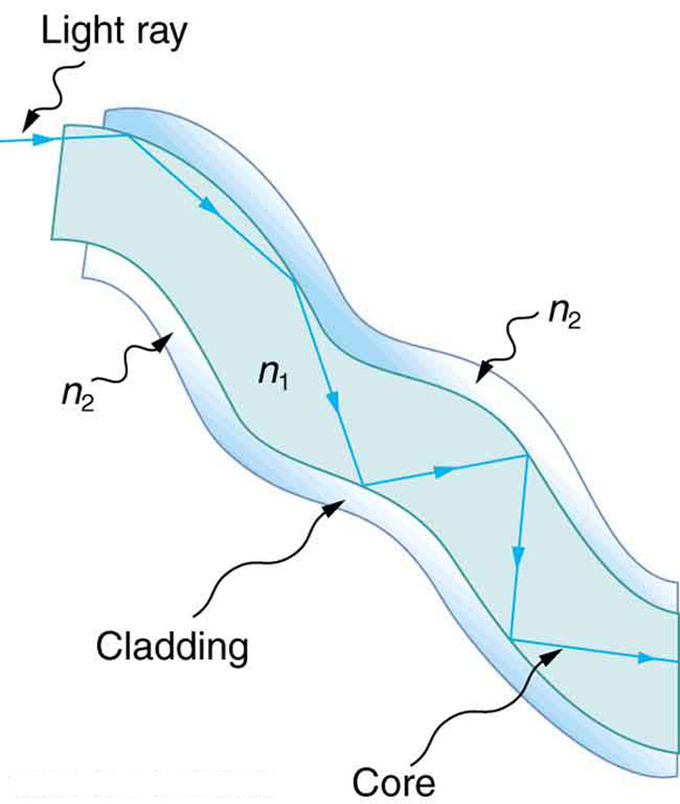Fig 2: Fibers in bundles are clad by a material that has a lower index of refraction than the core to ensure total internal reflection, even when fibers are in contact with one another. This shows a single fiber with its cladding.

The basic functional structure of an optical fiber consists of an outer protective cladding and an inner core through which light pulses travel. The overall diameter of the fiber is about 125 μm and that of the core is just about 50 μm. The difference in refractive index of the cladding and the core allows total internal reflection in the same way as happens at an air-water surface show in. If light is incident on a cable end with an angle of incidence greater than the critical angle then the light will remain trapped inside the glass strand. In this way, light travels very quickly down the length of the cable over a very long distance (tens of kilometers). Optical fibers are commonly used in telecommunications, because information can be transported over long distances, with minimal loss of data. Another common use can be found in medicine in endoscopes. The field of applied science and engineering concerned with the design and application of optical fibers are called fiber optics.

## Total Polarization

Brewster’s angle is an angle of incidence at which light with a particular polarization is perfectly transmitted through a surface.

### Learning Objectives

Calculate the Brewster’s angle from the indices of refraction and discuss its physical mechanism

### Key Takeaways

#### Key Points

• When light hits a surface at a Brewster angle, reflected beam is linearly polarized.
• The physical mechanism for the Brewster’s angle can be qualitatively understood from the manner in which electric dipoles in the media respond to p-polarized light.
• Brewsters’ angle is given as $\theta_\mathrm {\text{B}} = \arctan {\left( \frac{\text{n}_2}{\text{n}_1} \right)}$.

#### Key Terms

• dipole: A separation of positive and negative charges.
• dielectric: An electrically insulating or nonconducting material considered for its electric susceptibility (i.e., its property of polarization when exposed to an external electric field).
• polarizer: An optical filter that passes light of a specific polarization and blocks waves of other polarizations.

Brewster’s angle (also known as the polarization angle) is an angle of incidence at which light with a particular polarization is perfectly transmitted through a transparent dielectric surface, with no reflection. When unpolarized light is incident at this angle, the light that is reflected from the surface is therefore perfectly polarized. This special angle of incidence is named after the Scottish physicist Sir David Brewster (1781–1868).

The physical mechanism for this can be qualitatively understood from the manner in which electric dipoles in the media respond to p-polarized light (whose electric field is polarized in the same plane as the incident ray and the surface normal). One can imagine that light incident on the surface is absorbed, and then re-radiated by oscillating electric dipoles at the interface between the two media. The refracted light is emitted perpendicular to the direction of the dipole moment; no energy can be radiated in the direction of the dipole moment. Thus, if the angle of reflection θ1 (angle of reflection) is equal to the alignment of the dipoles (90 – θ2), where θ2 is angle of refraction, no light is reflected.Fig 1: An illustration of the polarization of light that is incident on an interface at Brewster’s angle.

This geometric condition can be expressed as $\theta_1 + \theta_2 = 90 ^{\circ}$, where θ1 is the angle of incidence and θ2 is the angle of refraction. Using Snell’s law (n1sinθ1 = n2sinθ2), one can calculate the incident angle θ1 = B at which no light is reflected: $\text{n}_1 \sin {\left( \theta_\mathrm {\text{B}} \right)} =\text{n}_2 \sin {\left( 90^\circ - \theta_\mathrm {\text{B}} \right)}=\text{n}_2 \cos {\left( \theta_\mathrm {\text{B}} \right)}.$Solving for θB gives $\theta_\mathrm {\text{B}} = \arctan {\left( \frac{\text{n}_2}{\text{n}_1} \right)}.$

When light hits a surface at a Brewster angle, reflected beam is linearly polarized. shows an example, where the reflected beam was nearly perfectly polarized and hence, blocked by a polarizer on the right picture. Polarized sunglasses use the same principle to reduce glare from the sun reflecting off horizontal surfaces such as water or road.Fig 2: Photograph taken of a window with a camera polarizer filter rotated to two different angles. In the picture at left, the polarizer is aligned with the polarization angle of the window reflection. In the picture at right, the polarizer has been rotated 90° eliminating the heavily polarized reflected sunlight.

Polarization Experience: A polarizing filter allows light of a particular plane of polarization to pass, but scatters the rest of the light. When two polarizing filters are crossed, almost no light gets through. Some materials have molecules that rotate the plane of polarization of light. When one of these materials is placed between crossed polarizing filters, more light is allowed to pass through.

## Dispersion: Rainbows and Prisims

Dispersion is defined as the spreading of white light into its full spectrum of wavelengths.

### Learning Objectives

Describe production of rainbows by a combination of refraction and reflection processes

### Key Takeaways

#### Key Points

• Dispersion occurs whenever there is a process that changes the direction of light in a manner that depends on wavelength. Dispersion can occur for any type of wave and always involves wavelength-dependent processes.
• For a given medium, n increases as wavelength decreases and is greatest for violet light. Thus violet light is bent more than red light, as can be seen with a prism.
• In a rainbow, light enters a drop of water and is reflected from the back of the drop. The light is refracted both as it enters and as it leaves the drop.

#### Key Terms

• refraction: Changing of a light ray’s direction when it passes through variations in matter.

We see about six colors in a rainbow—red, orange, yellow, green, blue, and violet; sometimes indigo is listed, too. These colors are associated with different wavelengths of light. White light, in particular, is a fairly uniform mixture of all visible wavelengths. Sunlight, considered to be white, actually appears to be a bit yellow because of its mixture of wavelengths, but it does contain all visible wavelengths. The sequence of colors in rainbows is the same sequence as the colors plotted versus wavelength. What this implies is that white light is spread out according to wavelength in a rainbow. Dispersion is defined as the spreading of white light into its full spectrum of wavelengths. More technically, dispersion occurs whenever there is a process that changes the direction of light in a manner that depends on wavelength. Dispersion, as a general phenomenon, can occur for any type of wave and always involves wavelength-dependent processes.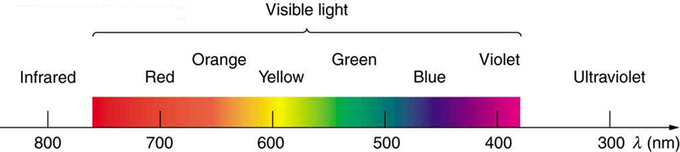Colors of a Rainbow: Even though rainbows are associated with seven colors, the rainbow is a continuous distribution of colors according to wavelengths.

Refraction is responsible for dispersion in rainbows and many other situations. The angle of refraction depends on the index of refraction, as we saw in the Law of Refraction. We know that the index of refraction n depends on the medium. But for a given medium, n also depends on wavelength. Note that, for a given medium, n increases as wavelength decreases and is greatest for violet light. Thus violet light is bent more than red light and the light is dispersed into the same sequence of wavelengths.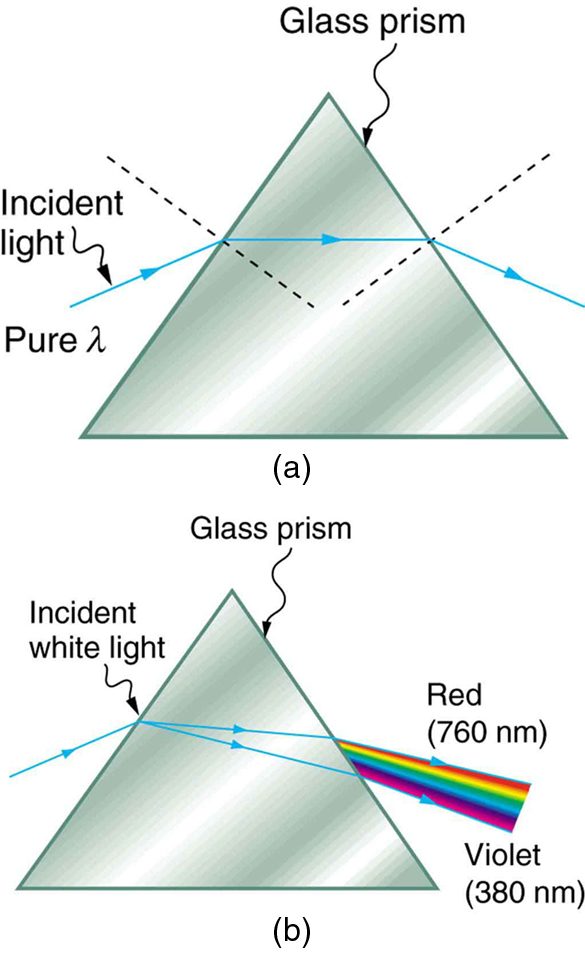Pure Light and Light Dispersion: (a) A pure wavelength of light falls onto a prism and is refracted at both surfaces. (b) White light is dispersed by the prism (shown exaggerated). Since the index of refraction varies with wavelength, the angles of refraction vary with wavelength. A sequence of red to violet is produced, because the index of refraction increases steadily with decreasing wavelength.

Rainbows are produced by a combination of refraction and reflection. You may have noticed that you see a rainbow only when you look away from the sun. Light enters a drop of water and is reflected from the back of the drop. The light is refracted both as it enters and as it leaves the drop. Since the index of refraction of water varies with wavelength, the light is dispersed, and a rainbow is observed. (There is no dispersion caused by reflection at the back surface, since the law of reflection does not depend on wavelength. ) The actual rainbow of colors seen by an observer depends on the myriad of rays being refracted and reflected toward the observer’s eyes from numerous drops of water. The arc of a rainbow comes from the need to be looking at a specific angle relative to the direction of the sun.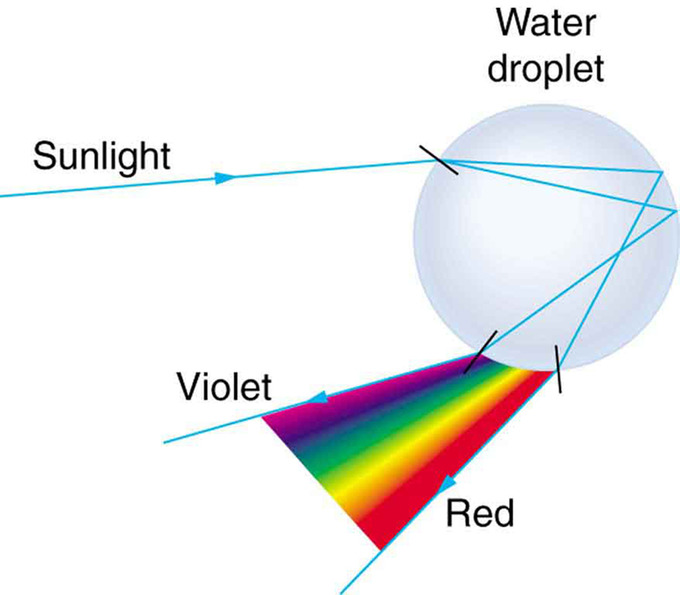Light Reflecting on Water Droplet: Part of the light falling on this water drop enters and is reflected from the back of the drop. This light is refracted and dispersed both as it enters and as it leaves the drop.